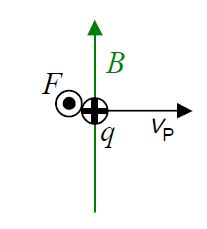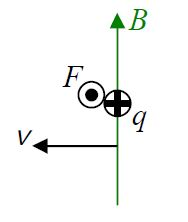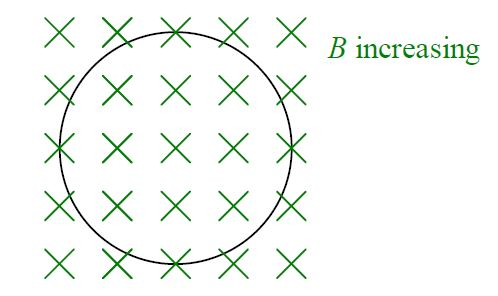$$\require{cancel}$$

# B37: Maxwell’s Equations

In this chapter, the plan is to summarize much of what we know about electricity and magnetism in a manner similar to the way in which James Clerk Maxwell summarized what was known about electricity and magnetism near the end of the nineteenth century. Maxwell not only organized and summarized what was known, but he added to the knowledge. From his work, we have a set of equations known as Maxwell’s Equations. His work culminated in the discovery that light is electromagnetic waves.

In building up to a presentation of Maxwell’s Equations, I first want to revisit ideas we encountered in chapter 20 and I want to start that revisit by introducing an easy way of relating the direction in which light is traveling to the directions of the electric and magnetic fields that are the light.

Recall the idea that a charged particle moving in a stationary magnetic fieldexperiences a force given by

$\vec{F}=q\vec{V}_p \times \vec{B}$

This force, by the way, is called the Lorentz Force. For the case depicted above, by the righthand rule for the cross product of two vectors, this force would be directed out of the page.Viewing the exact same situation from the reference frame in which the charged particle is at rest we see a magnetic field moving sideways (with velocity $$\vec{v}=-\vec{v}_p$$ ) through the particle. Since we have changed nothing but our viewpoint, the particle is experiencing the same force.We introduce a “middleman” by adopting the attitude that the moving magnetic field doesn’t really exert a force on the charged particle, rather it causes an electric field which does that. For the force to be accounted for by this middleman electric field, the latter must be in the direction of the force. The existence of light indicates that the electric field is caused to exist whether or not there is a charged particle for it to exert a force on.The bottom line is that wherever you have a magnetic field vector moving sideways through space you have an electric field vector, and, the direction of the velocity of the magnetic field vector is consistent with

$\mbox{direction of} \, \vec{V}=\mbox{direction of} \space \vec{E}\times \vec{B}.$

You arrive at the same result for the case of an electric field moving sideways through space. (Recall that in chapter 20, we discussed the fact that an electric field moving sideways through space causes a magnetic field.)

The purpose of this brief review of material from chapter 20 was to arrive at the result $$\mbox{direction of} \, \vec{V}=\mbox{direction of} \space \vec{E}\times \vec{B}$$. This direction relation will come in handy in our discussion of two of the four equations known as Maxwell’s Equations.

One of Maxwell’s Equations is called Faraday’s Law. It brings together a couple of things we have already talked about, namely, the idea that a changing number of magnetic field lines through a loop or a coil induces a current in that loop or coil, and, the idea that a magnetic field vector that is moving sideways through a point in space causes an electric field to exist at that point in space. The former is a manifestation of the latter. For instance, suppose you have an increasing number of downward directed magnetic field lines through a horizontal loop. The idea is that for the number of magnetic field lines through the loop to be increasing, there must be magnetic field lines moving sideways through the conducting material of the loop (to get inside the perimeter of the loop). This causes an electric field in the conducting material of the loop which in turn pushes on the charged particles of the conducting material of the loop and thus results in a current in the loop. We can discuss the production of the electric field at points in space occupied by the conducting loop even if the conducting loop is not there. If we consider an imaginary loop in its place, the magnetic field lines moving through it to the interior of the loop still produce an electric field in the loop; there are simply no charges for that field to push around the loop.

Suppose we have an increasing number of downward-directed magnetic field lines through an imaginary loop. Viewed from above the situation appears as:The big idea here is that you can’t have an increasing number of downward-directed magnetic field lines through the region encircled by the imaginary loop without having, either, downward directed magnetic field lines moving transversely and inward through the loop into the region encircled by the loop, or, upward-directed magnetic field lines moving transversely and outward through the loop out of the region encircled by the loop. Either way you have magnetic field lines cutting through the loop and with each magnetic field cutting through the loop there has to be an associated electric field with a component tangent to the loop. Our technical expression for the “number of magnetic field lines through the loop” is the magnetic flux, given, in the case of a uniform (but time-varying) magnetic field by

$\Phi_B=\vec{B}\cdot\vec{A}$

where A is the area of the region encircled by the loop.

Faraday’s Law, as it appears in Maxwell’s Equations, is a relation between the rate of change of the magnetic flux through the loop and the electric field (produced by this changing flux) in the loop. To arrive at it, we consider an infinitesimal segment $$dl$$ of the loop and the infinitesimal contribution to the rate of change of the magnetic flux through the loop resulting from magnetic field lines moving through that segment $$dl$$ into the region encircled by the loop.If the magnetic field depicted above is moving sideways toward the interior of the loop with a speed $$v=\frac{dx}{dt}$$ then all the magnetic field lines in the region of area $$A=dl\space dx$$, will, in time $$dt$$, move leftward a distance $$dx$$. That is, they will all move from outside the loop to inside the loop creating a change of flux, in time $$dt$$, of

$d\phi_B=B\space dA$

$d\phi_B=B\space dl\space dx$

Now, if I divide both sides of this equation by the time $$dt$$ in which the change occurs, we have

$\frac{d\phi_B}{dt}=B\space dl \frac{dx}{dt}$

which I can write as

$\dot{\phi_B}=B\space dl\space v$

or

$\dot{\phi_B}=v\space \, B\space dl$

For the case at hand, looking at the diagram, we see that $$\vec{B}$$ and $$\vec{v}$$ are at right angles to each other so the magnitude of $$\vec{v}\times \vec{B}$$ is just $$vB$$. In that case, since $$\vec{E}=-\vec{v}\times \vec{B}$$ (from equation 20-1 with $$-\vec{v}$$ in place of $$\vec{v}_P$$), we have $$E=vB$$. Replacing the product $$vB$$ appearing on the right side of equation 37-1 $$(\dot{phi}_B=vB\space dl)$$ yields $$\dot{\phi}_B=Edl$$ which I copy at the top of the following page:

$\dot{\phi}_B=Edl$

We can generalize this to the case where the velocity vector $$\vec{v}$$ is not perpendicular to the infinitesimal loop segment in which case $$\vec{E}$$ is not along $$\vec{dl}$$. In that case the component of $$\vec{E}$$ that is along $$\vec{dl}$$, times the length $$dl$$ itself, is just $$\vec{E}\cdot \vec{dl}$$ and our equation becomes

$\dot{\phi}_B=-\vec{E} \cdot \vec{dl}$

In this expression, the direction of $$\vec{dl}$$ is determined once one decides on which of the two directions in which a magnetic field line can extend through the region enclosed by the loop is defined to make a positive contribution to the flux through the loop. The direction of $$\vec{dl}$$ is then the one which relates the sense in which $$\vec{dl}$$ points around the loop, to the positive direction for magnetic field lines through the loop, by the right hand rule for something curly something straight. With this convention the minus sign is needed to make the dot product have the same sign as the sign of the ongoing change in flux. Consider for instance the case depicted in the diagram:We are looking at a horizontal loop from above. Downward is depicted as into the page. Calling downward the positive direction for flux makes clockwise, as viewed from above, the positive sense for the $$\vec{dl}$$’s in the loop, meaning the $$\vec{dl}$$ on the right side of the loop is pointing toward the bottom of the page (as depicted). For a downward-directed magnetic field moving leftward into the loop, $$\vec{E}$$ must be directed toward the top of the page (from $$\mbox{direction of}\space \vec{v}=\mbox{direction of}\space \vec{E}\times \vec{B}$$). Since $$\vec{E}$$ is in the opposite direction to that of $$\vec{dl}$$, $$\vec{E} \cdot \vec{dl}$$ must be negative. But movement of downward-directed magnetic field lines into the region encircled by the loop, what with downward being considered the positive direction for flux, means a positive rate of change of flux. The left side of $$\dot{\phi}_B=-\vec{E}\cdot \vec{dl}$$ is thus positive. With $$\vec{E}\cdot \vec{dl}$$ being negative, we need the minus sign in front of it to make the right side positive too. Now $$\dot{\phi}_B$$ is the rate of change of magnetic flux through the region encircled by the loop due to the magnetic field lines that are entering that region through the one infinitesimal $$\vec{dl}$$ that we have been considering. There is a $$\dot{\phi}_B$$ for each infinitesimal $$\vec{dl}$$ making up the loop. Thus there are an infinite number of them. Call the infinite sum of all the $$\dot{\phi}_B$$'s $$\dot{\Phi}_B$$ and our equation becomes: